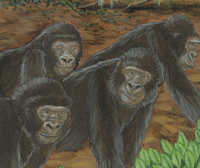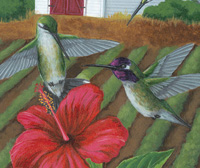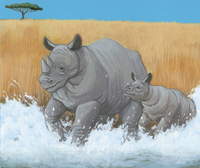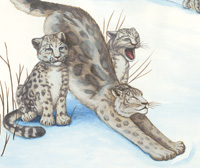Great Divide, The - For Creative Minds Quiz1. Both groups of llamas and groups of elephants are called ________.     Herds     Mobs     Smacks2. Whales live in rivers.     True     False3. Wolves live in which hemisphere?     Southern     Northern     Both4. Which animal lives in Antarctica?     Wolves     Elephants     Penguins5. What is a group of flamingos called?     A leap     A stand     A pod6. If you break a cookie in half and give one half to a friend, what mathematical operation did you just use?     Division     Multiplication     Addition7. Addition is the opposite of _________.     Multiplication     Division     Subtraction8. Multiplication is the opposite of _________.     Addition     Division     Subtraction9. What is the answer to a division problem called?     The sum     The dividend     The product10. In a division problem, it doesnâ€™t matter which divisor is used first, the answer will always be the other divisor.     True     False